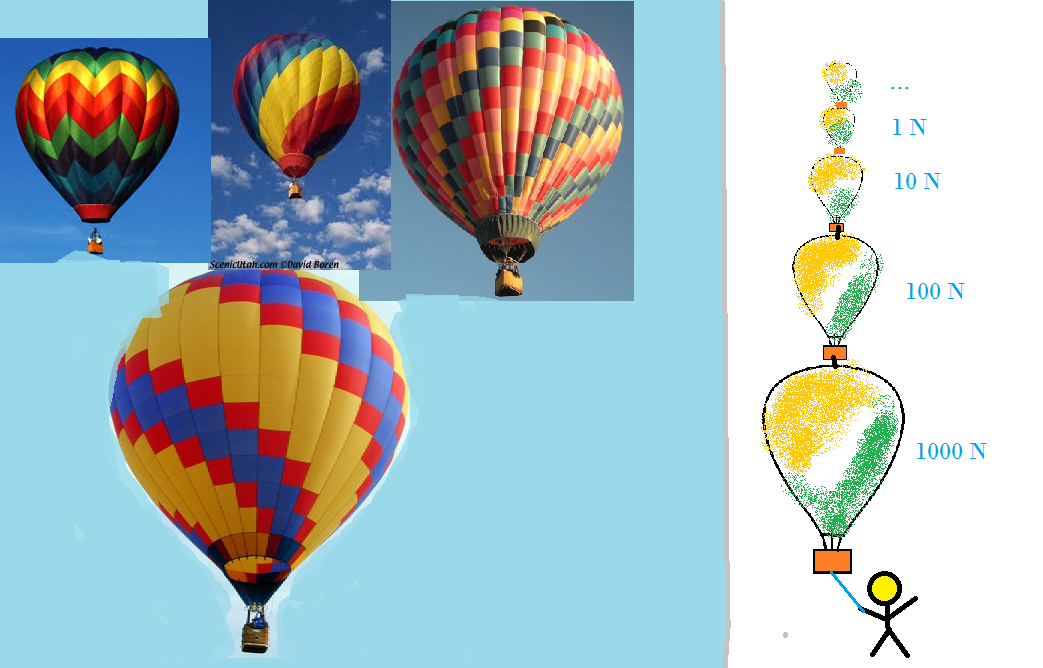# Cody's Awesome Balloons -2Cody (colored Yellow head) wants to fly up in the sky, so he has tied infinite hot air balloons to each other as shown in the right part of the image (Yeah, he can do that, infinite balloons ) .

The largest balloon is providing him an upward force of $1000$ N. The balloon just above that largest balloon is providing an additional force of $100$ N. The third provides $10$ N, and so on.

If Cody weighs $54.4$ kg, find with how much acceleration he is going up in the sky.

Details and assumptions:-

$\bullet$ $54.4$ kg is Cody's mass.

$\bullet$ Acceleration due to gravity $g=10 m/s^2$

$\bullet$ Cody is so awesome that he made balloons of really negligible mass.

This problem is a part of the set Cody Mechanics

×